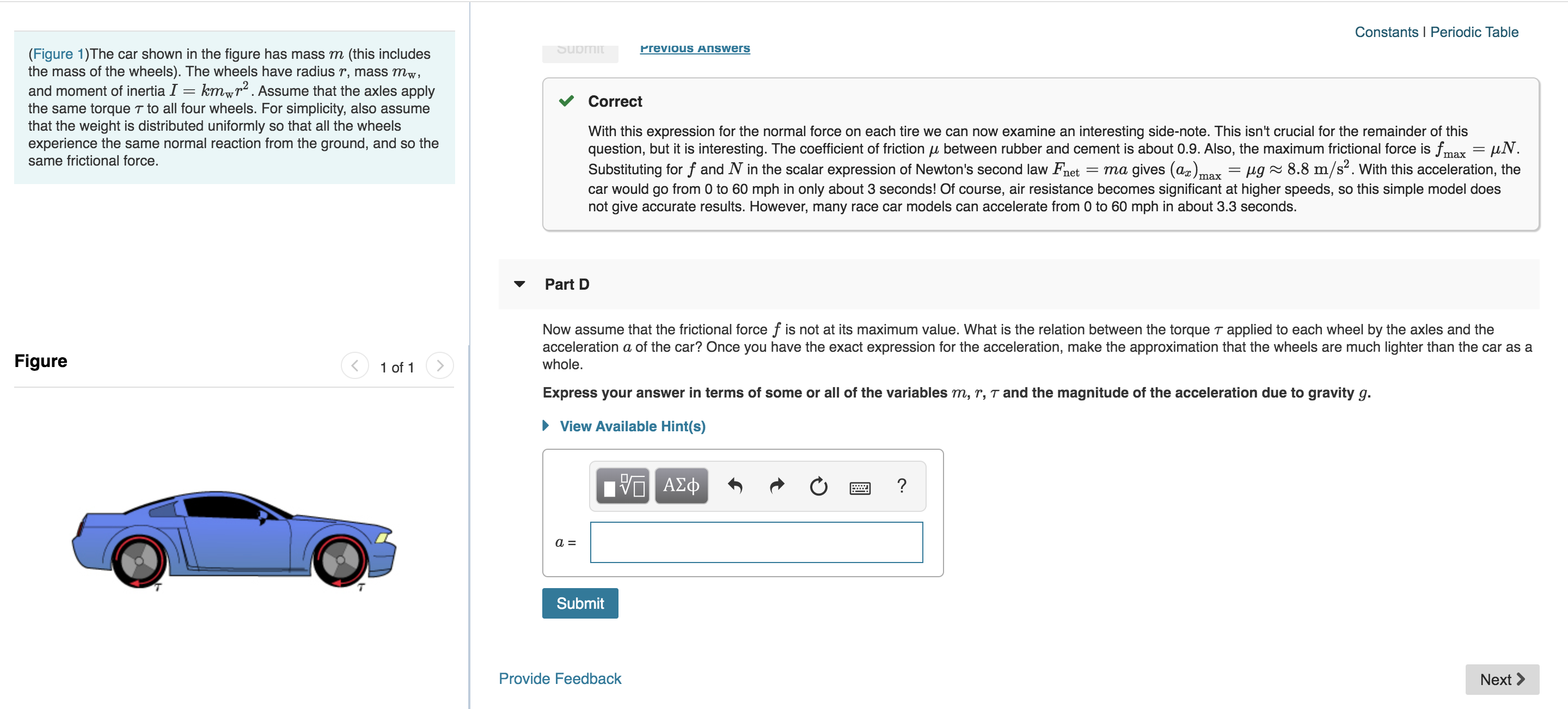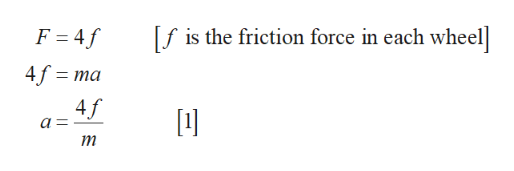# Constants I Periodic TableSubmitPrevious Answers(Figure 1)The car shown in the figure has mass m (this includesthe mass of the wheels). The wheels have radius r, mass mw,and moment of inertia I = kmwr2. Assume that the axles applythe same torque T to all four wheels. For simplicity, also assumethat the weight is distributed uniformly so that all the wheelsexperience the same normal reaction from the ground, and so thesame frictional force.CorrectWith this expression for the normal force on each tire we can now examine an interesting side-note. This isn't crucial for the remainder of thisquestion, but it is interesting. The coefficient of friction u between rubber and cement is about 0.9. Also, the maximum frictional force is fm = uNSubstituting for f and N in the scalar expression of Newton's second law Fnetcar would go from 0 to 60 mph in only about 3 seconds! Of course, air resistance becomes significant at higher speeds, so this simple model doesnot give accurate results. However, many race car models can accelerate from 0 to 60 mph in about 3.3 seconds.max= ma gives (a)max = /ug^ 8.8 m/s2. With this acceleration, thePart DNow assume that the frictional force f is not at its maximum value. What is the relation between the torque T applied to each wheel by the axles and theacceleration a of the car? Once you have the exact expression for the acceleration, make the approximation that the wheels are much lighter than the car as awhole.Figure1 of 1Express your answer in terms of some or all of the variables m, r, T and the magnitude of the acceleration due to gravity g.View Available Hint(s)ν ΑΣφ?SubmitProvide FeedbackNext

Question
80 views

Part Dhelp_outlineImage TranscriptioncloseConstants I Periodic Table Submit Previous Answers (Figure 1)The car shown in the figure has mass m (this includes the mass of the wheels). The wheels have radius r, mass mw, and moment of inertia I = kmwr2. Assume that the axles apply the same torque T to all four wheels. For simplicity, also assume that the weight is distributed uniformly so that all the wheels experience the same normal reaction from the ground, and so the same frictional force. Correct With this expression for the normal force on each tire we can now examine an interesting side-note. This isn't crucial for the remainder of this question, but it is interesting. The coefficient of friction u between rubber and cement is about 0.9. Also, the maximum frictional force is fm = uN Substituting for f and N in the scalar expression of Newton's second law Fnet car would go from 0 to 60 mph in only about 3 seconds! Of course, air resistance becomes significant at higher speeds, so this simple model does not give accurate results. However, many race car models can accelerate from 0 to 60 mph in about 3.3 seconds. max = ma gives (a)max = /ug^ 8.8 m/s2. With this acceleration, the Part D Now assume that the frictional force f is not at its maximum value. What is the relation between the torque T applied to each wheel by the axles and the acceleration a of the car? Once you have the exact expression for the acceleration, make the approximation that the wheels are much lighter than the car as a whole. Figure 1 of 1 Express your answer in terms of some or all of the variables m, r, T and the magnitude of the acceleration due to gravity g. View Available Hint(s) ν ΑΣφ ? Submit Provide Feedback Next fullscreen
check_circle

Step 1

Write the expression for net force in the vertical direction and equate with the Newton’s second law,help_outlineImage Transcriptionclosef is the friction force in each wheel F = 4f 4f ma 4f fullscreen
Step 2

Write the expression for torque in ...

### Want to see the full answer?

See Solution

#### Want to see this answer and more?

Solutions are written by subject experts who are available 24/7. Questions are typically answered within 1 hour.*

See Solution
*Response times may vary by subject and question.
Tagged in

### Physics